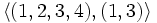# Cyclic four-subgroups of symmetric group:S4

This article is about a particular subgroup in a group, up to equivalence of subgroups (i.e., an isomorphism of groups that induces the corresponding isomorphism of subgroups). The subgroup is (up to isomorphism) cyclic group:Z4 and the group is (up to isomorphism) symmetric group:S4 (see subgroup structure of symmetric group:S4).
VIEW: Group-subgroup pairs with the same subgroup part | Group-subgroup pairs with the same group part | All pages on particular subgroups in groups

We denote the symmetric group$S_4$ on$\{ 1,2,3,4 \}$ by$G$. We define:$H := \langle (1,2,3,4) \rangle = \langle (1,4,3,2) \rangle = \{ (), (1,2,3,4), (1,3)(2,4), (1,4,3,2) \}$.

Its other images under inner automorphisms (which are the same as its images under automorphisms) are:

•$\langle (1,3,2,4) \rangle = \langle (1,4,2,3) \rangle = \{ (), (1,3,2,4), (1,2)(3,4), (1,4,2,3) \}$.
•$\langle (1,3,4,2) \rangle = \langle (1,2,4,3) \rangle = \{ (), (1,3,4,2), (1,4)(2,3), (1,2,4,3) \}$.

## Arithmetic functions

Function Value Explanation
order of whole group 24
order of subgroup 4
index 6
size of conjugacy class 3

## Effect of subgroup operators

Function Value as subgroup (descriptive) value as subgroup (link) Value as group
normalizer$\langle (1,2,3,4), (1,3) \rangle$ D8 in S4 dihedral group:D8
centralizer$\langle (1,2,3,4) \rangle$ same as$H$ cyclic group:Z4
normal core$()$ trivial subgroup trivial group
normal closure$G$ whole group symmetric group:S4

## Related subgroups

### Intermediate subgroups

Value of intermediate subgroup (descriptive) Isomorphism class of intermediate subgroup Small subgroup in intermediate subgroup Intermediate subgroup in big group$\langle (1,2,3,4), (1,3) \rangle$ dihedral group:D8 cyclic maximal subgroup of dihedral group:D8 D8 in S4

### Smaller subgroups

Value of smaller subgroup (descriptive) Isomorphism class of smaller subgroup Smaller subgroup in subgroup Smaller subgroup in whole group$\langle (1,3)(2,4) \rangle$ cyclic group:Z2 Z2 in Z4

## Resemblance-related properties satisfied

### Isomorph-conjugate subgroup

Further information: isomorph-conjugate subgroup, isomorph-automorphic subgroup, automorph-conjugate subgroup$H$ is an isomorph-conjugate subgroup of$G$: it is conjugate to all the other subgroups isomorphic to it. Hence, it is also an isomorph-automorphic subgroup and an automorph-conjugate subgroup. Note that since$G$ is a complete group (symmetric groups are complete) all subgroups of$G$ are automorph-conjugate.

### Subgroup whose join with any distinct conjugate is the whole group

Further information: subgroup whose join with any distinct conjugate is the whole group

The join of$H$ and any conjugate of$H$ distinct from$H$ is the whole group. In particular, this forces that:

## Opposites of normality satisfied and dissatisfied

### Opposites satisfied

•$H$ is a core-free subgroup of$G$: the normal core of$H$ in$G$ is trivial.
•$H$ is a contranormal subgroup of$G$: the normal closure of$H$ in$G$ is$G$.

### Opposites dissatisfied

•$H$ is not a self-normalizing subgroup of$G$.
•$H$ is not an abnormal subgroup of$G$ or a weakly abnormal subgroup of$G$, because both of these would imply that$H$ is a self-normalizing subgroup of$G$.